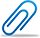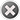Cash-back offer from 1st to 8th October 2023. Get flat 10% cash-back credited to your account for a minimum transaction of \$50. Post Your Questions Today!

Question Details Normal
\$ 50.00

# IEOR 3402: Assignment 4 help needed

• From Mathematics, Statistics
• Due on 19 Feb, 2015 09:12:00
• Asked On 16 Feb, 2015 09:46:56
• Due date has already passed, but you can still post solutions.
Question posted byIEOR 3402: Assignment 4

1. Nahmias 4.10.

2. Quarter-inch stainless-steel bolts are consumed in a factory at a fairly steady rate of 60 per week. The bolts cost the plant two cents each. It costs the plant \$12 to initiate an order, and holding costs are based on an annual interest rate of 25 percent.
(a) Determine the optimal number of bolts for the plant to purchase and the time between placements of orders.
(b) What is the yearly holding and setup cost for this item?

3. Reconsider the bolt example in Problem 2. Suppose that although we have estimated demand to be 60 per week, it turns out that it is actually 120 per week (i.e., we have a 100 percent forecasting error).
(a) If we use the lot size calculated in the previous problem (i.e., using the erroneous demand estimate), what will the setup plus holding cost be under the true demand rate?
(b) What would the cost be if we had used the optimum lot size?
(c) What percentage increase in cost was caused by the 100 percent demand forecasting error? What does this tell you about the sensitivity of the EOQ model to errors in the data?

4. Consider the bolt example in Problem 2, assuming that the demand of 60 per week is correct. Now, however, suppose the minimum reorder interval is one month and all order cycles are placed on a power-of-two multiple of months (that is, one month, two months, four months, eight months, etc) in order to permit truck sharing with orders of other parts.
(a) What is the least-cost reorder interval under this restriction?
(b) How much does this add to the total cost?
(c) How is the effectiveness of powers-of-2 order intervals related to the result of the previous problem regarding the effect of demand forecasting errors?

5. * Nahmias, 4.17.

6. * In the EOQ model with planned backorders, suppose the parameters are λ = 1000, K = 60, h =0.75 and b =0.81. Compute the optimal policy and the optimal average cost (excluding cλ).

7. Nahmias, 4.26.

8. * Nahmias, 4.30.Attachment
\$ 50.00

## [Solved] IEOR 3402: Assignment 4 Complete Solution

• This Solution has been Purchased 1 time
• Submitted On 16 Feb, 2015 09:50:02We know that cost function in the EOQ model has a s...

Buy now to view the complete solutionOther Related Questions

No related question exists

#### The benefits of buying study notes from CourseMerits##### Assurance Of Timely Delivery
We value your patience, and to ensure you always receive your homework help within the promised time, our dedicated team of tutors begins their work as soon as the request arrives.##### Best Price In The Market
All the services that are available on our page cost only a nominal amount of money. In fact, the prices are lower than the industry standards. You can always expect value for money from us.